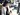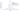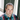# Introduction to mass spectrometry data analysis

##### This post is part of our series titled "Mass spectrometry data introduction", which contains the following entries:Introduction to mass spectrometry data analysis (current post)Introduction to mass spectrometry imaging data analysis

• Anatomy of a mass spectrometer
• Output of MS experiments: the glorious mass spectrum
• Challenges in analyzing MS data
• Conclusion

• ## Anatomy of a mass spectrometer

##### A mass spectrometer involves at least three components:
1. An ionization source, which is responsible for generating gas-phase ions from the sample. These ions can then be manipulated using electric and/or magnetic fields. Generating ions can be done in many ways, for instance using laser desorption (LDI) or electrospray (ESI).
2. A mass analyzer, which sorts and separates the ions generated in the source based on their m/z values. A straightforward approach is using so-called time-of-flight, which consists of a linear path ions must travel under a constant electrical field. When two ions have the same charge, the ion with the higher mass will take longer to close the distance.
3. An ion detector which measures the relative abundance of ions separated by the mass analyzer, stratified over the different mass-to-charge ratios (m/z values).

## Output of MS experiments: the glorious mass spectrum

##### Once our trusty mass spectrometer has finished generating, sorting and counting ions, a mass spectrum is generated based on our sample. A mass spectrum is essentially a histogram spanning the m/z values that were measured. The intensity of a peak at a given m/z value indicates the relative frequency of detected ions with that specific m/z value within the sample.## Challenges in analyzing MS data

### Interpreting spectra

##### Second, it is important to know that there is usually no direct way to unambiguously link a single peak (i.e., 1 m/z value) to a specific biomolecular ion, and vice versa. If you are not familiar with mass spectrometry this may sound counterintuitive. The reason for this is twofold:
1. One peak could indicate several biomolecular ions, for several reasons:
• Isomers are organic compounds with the same molecular formula (= same mass) but different structures, and may have completely different chemical properties. A common example are cis and trans fatty acids.
• Distinct molecules may be closer to eachother in mass-to-charge ratio than the mass spectrometer can distinguish, i.e., slightly different m/z values ending up in the same bin. This occurs more frequently in low mass resolution instruments.
2. One molecule shows expression at multiple m/z values due to adduct formation and isotopical peaks. Isotopes are atom species of the same chemical element that have different masses, caused by a different number of neutrons. Examples include 1H and 2H or 12C and 13C. A single molecule with a given elemental formula will therefore give rise to an isotopic distribution, i.e., a set of peaks at different m/z values, the location and intensity distribution of which are determined by its constituent atoms.## Conclusion

##### This post is part of our series titled "Mass spectrometry data introduction", which contains the following entries:Introduction to mass spectrometry data analysis (current post)Introduction to mass spectrometry imaging data analysis